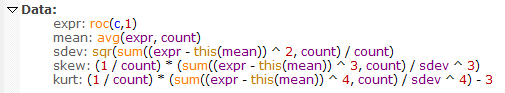Navigation: Realtest Script Language > Syntax Element Details > Kurtosis

Category

Multi-Bar Functions

Description

Statistical measure of the heaviness of the tail of a distribution of values

Syntax

Kurtosis(expr, count)

Parameters

expr - data series formula

count - lookback period

Notes

Kurtosis is calculated in the way that Excel would calculate a KURT.P function if it had one, i.e., as if the set of count values is the entire population.

The specific formula used is shown below, in the "kurt" item:This also illustrates how these statistical functions could be calculated in the Data Section of a script, though since they're provided built-in, there's no reason to do so.

This function supports ultra-fast one-pass calculation when used in the Data Section with a non-variable count.